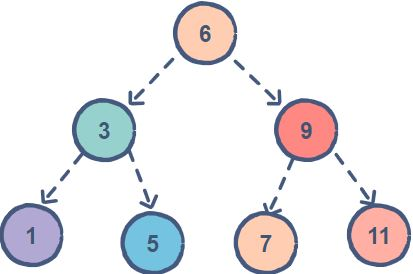Related Tags

# How to check if a binary tree is a binary search treeEducative Answers Team

A Binary Search Tree (BST) is a binary tree with the following properties:

• The left subtree of a particular node will always contain nodes whose keys are less than that node’s key.

• The right subtree of a particular node will always contain nodes with keys greater than that node’s key.

• The left and right subtree of a particular node will also, in turn, be binary search trees.

The tree on the right is a BST.## Algorithm

To see if a binary tree is a binary search tree, check:

1. If a node is a left child, then its key and the keys of the nodes in its right subtree are less than its parent’s key.

2. If a node is a right child, then its key and the keys of the nodes in its left subtree are greater than its parent’s key.

## Code

// Including dependancies.
#include <iostream>
#include <limits>
using namespace std;

// A node of a tree.
struct Tree
{
int data;
Tree *left;
Tree *right;
Tree(int data)
{
this -> data = data;
left = NULL;
right = NULL;
}
};

// Function to check if a binary tree is a BST. Note that this
// code allows for duplicate keys.
bool isBST(Tree* node, int min, int max) {
// Base case. An empty tree is a BST.
if (node == NULL)
return true;
// Checking if a key is outside the permitted range.
if (node -> data <= min)
return false;
if (node -> data >= max)
return false;
// Sending in updates ranges to the right and left subtree
return isBST(node -> right, node -> data, max) &&
isBST(node -> left, min, node -> data);
}

int main() {
// Creating a BST
Tree *root = new Tree(6);
root -> left = new Tree(3);
root -> right = new Tree(9);
root -> left -> left = new Tree(1);
root -> left -> right = new Tree(5);
root -> right -> left = new Tree(7);
root -> right -> right = new Tree(11);
// Passing in the min and the max value that can be
// represented inside an integer.
int min = std::numeric_limits<int>::min();
int max = std::numeric_limits<int>::max();
if(isBST(root, min, max)){
cout<<"This binary tree is a BST."<<endl;
}
else{
cout<<"This binary tree is not a BST."<<endl;
}
return 0;
}

RELATED TAGS